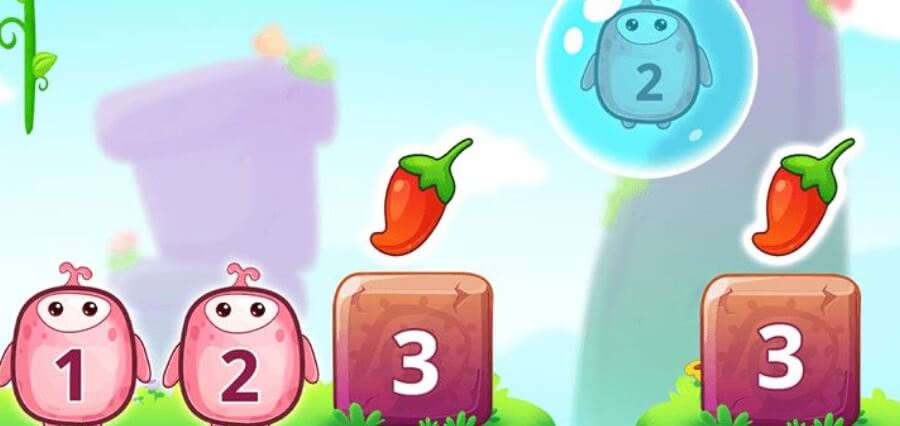# Here Are 5 Fun Online Games For Practising Math

Math subjects are generally not on the lists of most students. Learning math is seen as the most tedious task. Some teachers and parents find it challenging to teach math to students. Be it any part of math, like geometry, algebra, or even simple arithmetic. Teaching math for children in a traditional method is quite boring sometimes. Now here comes the solution to the problem. If traditional methods are not working, why not switch to a modern method?

The modern method is to study math online, which broadly includes games and other fun activities. Math games are always engaging in nature. There are so many math games that will add fun elements while learning. A few of them are mentioned below.

Bingo

Originally, the Bingo game was different. But teachers or parents can make slight alterations to this game. Here in this Bingo, teachers can start playing games by distributing the cards among the students with the product of the numbers written on them. The instruction to be given to the students is that the teacher will call out a multiplication equation, and the product of that equation will be printed on the cards. Students will analyse the equation and check their cards to see if the result is printed on their cards. If the equation matches the product on their cards, they can shout, “BINGO!”

Twin-Towers- An odd and even

Here the teacher will give the students in an online class a set of playing cubes. She can give a time limit of 1 minute and ask the students to build two towers of these snap cubes, one after the other. After the time is up, she’ll make them count the cubes in each tower and tell them which is the odd number and which is the even number.

Or another trick to learning odd-even is to see which tower is longer than the others. That will be the odd tower.

Find the Sum: An Addition-Based Learning Game

In this game, a teacher can either create a game worksheet or an online mode would be better. In this game, the teacher will write a sum in a question and provide some numbers in a box below, and ask the students which two or three numbers will add to give the sum given above. Let us see an example of it:

The teacher will give the following numbers to students:

46, 39, 20: 43, and ask them to add which two numbers will give the sum of 89.

The answers would be 46 and 43.

Number Nuts

Number Nuts is a set of brainy games. These games can be played by students on their own, without any classroom activity. The students will get an idea of the game type when they visit the website’s homepage. They can choose the best quiz type for themselves and start playing it.

Toy Theatre

Toy Theatre is another fantastic math game that teaches students other dimensions of math, such as patterns, counting, less than, greater than, and or equal to questions using simple yet appealing graphic representations.

## Conclusion

To sum up, apart from the games mentioned above, endless math games are available online that can help boost your child’s engagement in the subject.